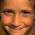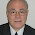## Thursday, April 23, 2009

### Confusion about nonlinearities in biochemical networks

In a recent discussion I was temporally confused about the question of whether the covalent modification networkis linear or not.

(I admit that this already has made me think about the possibility of nonlinear behavior emerging as an artifact of a coarse-grained description of a fundamentally linear dynamic system.
)

In this post I want to clarify this point.

The following figure shows an arbitrary example of a biochemical reaction network:The letters stand for chemical species and at the same time for the dynamical variables describing the temporally changing concentrations of these species. Here, X is an "input chemical", i.e. its concentration is completely under control of the experimenter. The concentration of the "output chemical" Y is measured by the experimenter, without disturbing its value. Inside the reaction network there can be an arbitrary number of other species z_n, dynamically coupled by chemical reactions. Note that we describe the system, from the outside, by only one input and one output variable, although the internal dynamical state has more dimensions.

We consider y(t) as a general functional of the input x(t):
y(t) = F\left\{x(t)\right\}.

If the reaction network would be a linear system, the functional could be written as a convolution with a Greensfunction:
y(t) = \int_{-\infty}^t G(t-t^{\prime}) x(t^{\prime}) dt^{\prime}.

In a mass action approximation, the temporal change of the various chemicals is described by coupled first order differential equations:
\frac{d}{dt}y = f_y(x,y,\vec{z})

and
\frac{d}{dt}\vec{z} = f_z(x,y,\vec{z}).

Here, the vector z stands for all the interior chemicals of the network.

The only case where we can actually define a Greensfunction is the trivial case where the right hand sides of the above equations are simple linear combinations of the variables:
f_y(x,y,\vec{z})=a\;x+b\;y+\vec{c}\;\vec{z}

f_z(x,y,\vec{z})=d\;x+e\;y+\vec{g}\;\vec{z}.

Here, the quantities a-g stand for fixed coefficients. More complicated terms such as products of variables are not permitted.

What does this mean for the structure of biochemical reaction networks ? We must not have any bi- (or higher-) molecular reactions involved. For example, in the above example network, the equation for the temporal change of Z2 reads
\frac{d}{dt}\vec{z_2} = k_1\; x\; z_1 - k_2\; z_2.

The " x * z1 " term already makes the system nonlinear.

The covalent modification network also contains bi-molecular reactions (where the enzyme-substrate complexes are formed). It is therefore nonlinear.

In conclusion: There are no multi-molecular reactions in a linear network.

#### 3 comments:

1.no one is ever going to write a comment as long this requires the effort to type a word verification and to sign up to a Google account. If you allow for anyone to publish his or her comments, you might get more feedback

2.Hi "Maximilian", that was a very good hint - THANKS ! I have changed the settings now.

3.Interesting observations that strike at the heart of chemical and biological complexity. There may be other ways to model nonlinear systems: See -
http://www.bio-balance.com/Weber%27s_Law.pdf